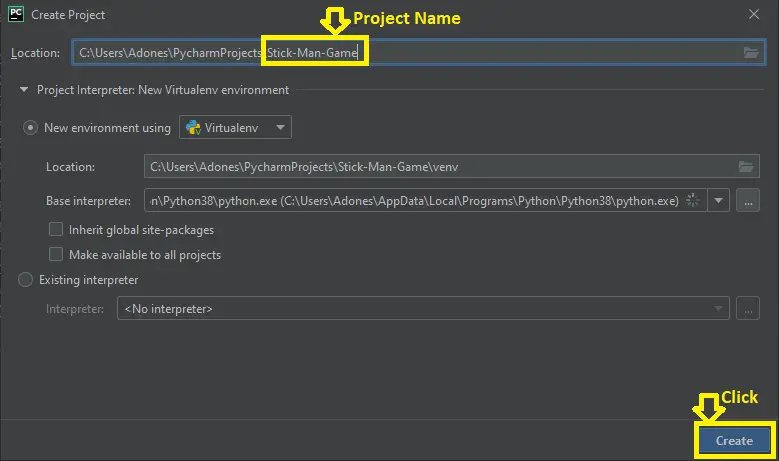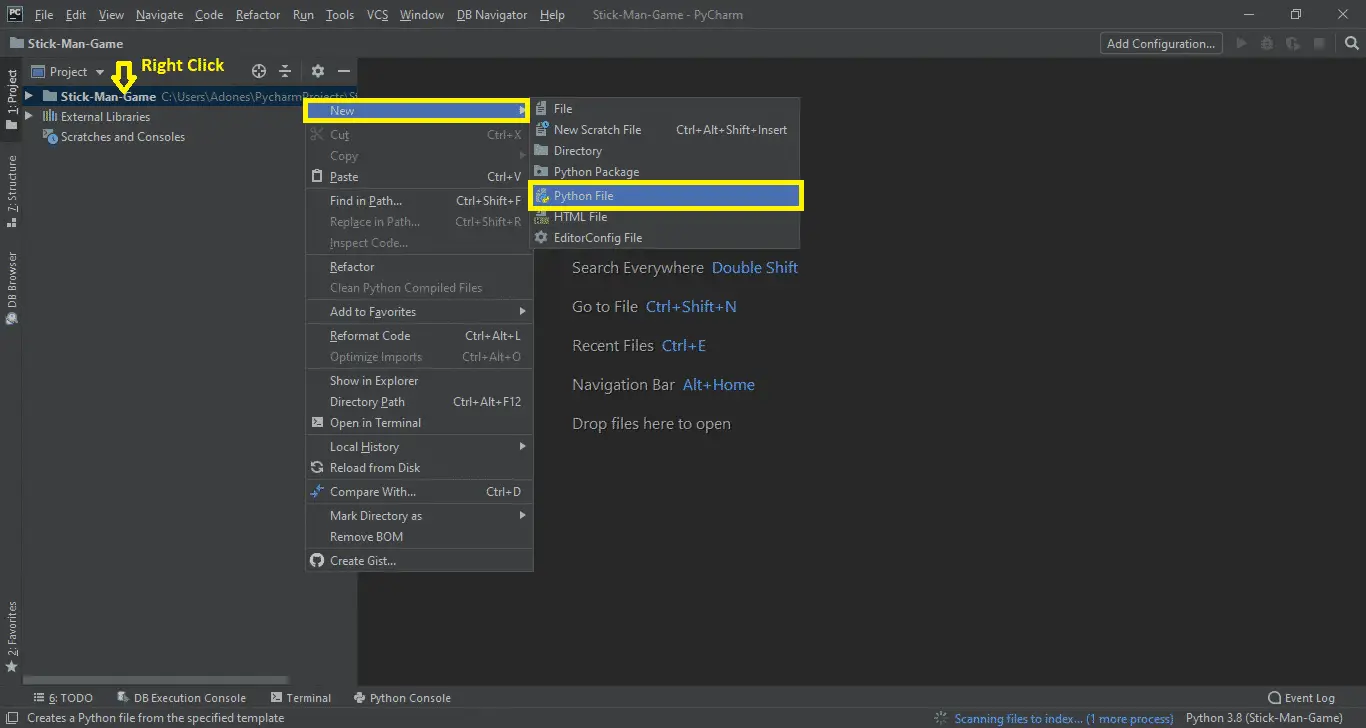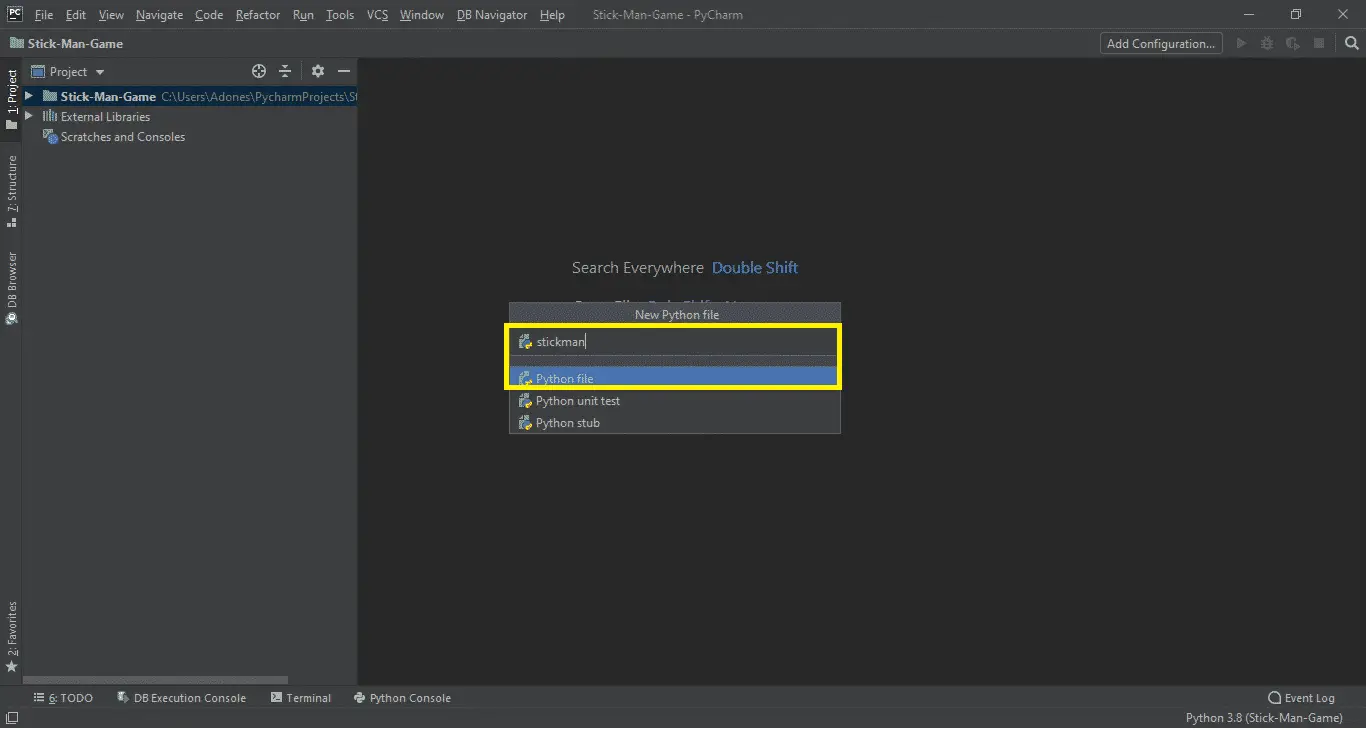# Stickman Game in Python with Source Code

## Stickman Game in Python with Source Code

Stickman Game in Python is created using tkinter and graphical user interface. The Stickman Game is developed in python programming language and it is a desktop application. This stickman game project contains a python script(stickman.py) and resources files. The graphics of a game is excellent and the controls are easy for the users to used.

This Stickman Game process are too easy all you need to do is attain to the go out into the end point. Here, the player has to leap via unique bars and on the pinnacle, and go out to the door.

The player hold on going for walks at a certain speed each time the participant press left or right arrow keys. Until and unless, the stickman receives caught through an item or wall, the participant continues on moving.

The predominant goal of this game is to attain the pinnacle stage to go out. The gamer has to use left and right arrow keys to move around the stickman and for the use of space bar is to jump the stickman.

Anyway if you want level up your knowledge in programming especially games in python, try this new article I’ve made for you Code For Game in Python: Python Game Projects With Source Code

Before you start on how to create Stickman Game in Python, make sure that you have PyCharm IDE installed in your computer.

By the way if you are new to python programming and you don’t know what would be the the Python IDE to use, I have here a list of Best Python IDE for Windows, Linux, Mac OS that will suit for you. I also have here How to Download and Install Latest Version of Python on Windows.

## This are thesteps on How to Create Stickman Game in Python.

Stickman Game in Python with Source Code

• Step 1: Create a project name.

First when you finished installed the Pycharm IDE in your computer, open it and then create a “project name” after creating a project name click the “create” button.• Step 2: Create a python file.

Second after creating a project name, “right click” your project name and then click “new” after that click the “python file“.• Step 3: Name your python file.

Third after creating a python file, Name your python file after that click “enter“.• Step 4: The actual code.

This is the actual coding on how to create Stickman Game in Python, and you are free to copy this code and download the full source code given below.

## Importing Tkinter Module

In the code given, which is for the importing tkinter function. Tkinter is the standard GUI library for Python. Python when combined with Tkinter provides a fast and easy way to create GUI applications.

`from tkinter import *`

## This Module for the Design Main Screen Window

In the code given below, which is first of all you have to design the main screen window. This display screen have a title of a game, canvas and image background.(Stickman Game in Python)

```class Game:
def __init__(self):
self.tk = Tk()
self.tk.title("Stick Man -Brought to you by Itsourcecode.com")
self.tk.resizable(0, 0)
self.tk.wm_attributes("-topmost", 1)
self.canvas = Canvas(self.tk, width=500, height=500, highlightthickness=0)
self.canvas.pack()
self.tk.update()
self.canvas_height = 500
self.canvas_width = 500
self.bg = PhotoImage(file="resources/background.png")
w = self.bg.width()
h = self.bg.height()
for x in range(0, 5):
for y in range(0, 5):
self.canvas.create_image(x * w, y * h, image=self.bg, anchor='nw')
self.sprites = []
self.running = True
self.game_over_text = self.canvas.create_text(250, 250, text='YOU WIN!', font=('Times', 30), state='hidden', fill='red')
self.out = self.canvas.create_text(58, 22, text='EXIT', font=('Times', 7), fill='red')```

## This module is for the class coordinates function

In the code given below, which is for the class coordinates function of x and y which is the right, left, top and bottom.(Stickman Game in Python)

``` class Coordinates:
def __init__(self, x1=0, y1=0, x2=0, y2=0):
self.x1 = x1
self.y1 = y1
self.x2 = x2
self.y2 = y2

def within_x(c1, c2):
if (c1.x1 > c2.x1 and c1.x1 < c2.x2) or (c1.x2 > c2.x1 and c1.x2 < c2.x2) or (c2.x1 > c1.x1 and c2.x1 < c1.x2) or (c2.x2 > c1.x1 and c2.x2 < c1.x1):
return True
else:
return False

def within_y(c1, c2):
if (c1.y1 > c2.y1 and c1.y1 < c2.y2) or (c1.y2 > c2.y1 and c1.y2 < c2.y2) or (c2.y1 > c1.y1 and c2.y1 < c1.y2) or (c2.y2 > c1.y1 and c2.y2 < c1.y1):
return True
else:
return False

def collided_left(c1, c2):
if within_y(c1, c2):
if c1.x1 <= c2.x2 and c1.x1 >= c2.x1:
return True
return False

def collided_right(c1, c2):
if within_y(c1, c2):
if c1.x2 >= c2.x1 and c1.x2 <= c2.x2:
return True
return False

def collided_top(c1, c2):
if within_x(c1, c2):
if c1.y1 <= c2.y2 and c1.y1 >= c2.y1:
return True
return False

def collided_bottom(y, c1, c2):
if within_x(c1, c2):
y_calculation = c1.y2 + y
if y_calculation >= c2.y1 and y_calculation <= c2.y2:
return True
return False```

## This module is for the class door function

In the code given below, which is for the door function of open and close door and the photo image.(Stickman Game in Python)

```class DoorSprite(Sprite):
def __init__(self, g, x, y, width, height):#hei1353
Sprite.__init__(self, g)
self.closed_door = PhotoImage(file="resources/door1.gif")
self.open_door = PhotoImage(file="resources/door2.gif")
self.image = g.canvas.create_image(x, y, image=self.closed_door, anchor='nw')
self.coordinates = Coordinates(x, y, x + (width / 2), y + height)
self.endgame = True

def op_door(self):
self.game.canvas.itemconfig(self.image, image=self.open_door)
def cl_door(self):
self.game.canvas.itemconfig(self.image, image=self.closed_door)

## This module is for the class stickman

In the code given below, which is for the function of stickman use in a game. The stickman can move to turn left, turn right, and jump.(Stickman Game in Python)

```class StickFigureSprite(Sprite):
def __init__(self, g):
Sprite.__init__(self, g)
self.images_left = [
PhotoImage(file="resources/figure-L1.gif"),
PhotoImage(file="resources/figure-L2.gif"),
PhotoImage(file="resources/figure-L3.gif")
]
self.images_right = [
PhotoImage(file="resources/figure-R1.gif"),
PhotoImage(file="resources/figure-R2.gif"),
PhotoImage(file="resources/figure-R3.gif")
]
self.image = g.canvas.create_image(200, 470, \
image=self.images_left, anchor='nw')
self.x = -2
self.y = 0
self.current_image = 0
self.jump_count = 0
self.last_time = time.time()
self.coordinates = Coordinates()
g.canvas.bind_all('<KeyPress-Left>', self.turn_left)
g.canvas.bind_all('<KeyPress-Right>', self.turn_right)
g.canvas.bind_all('<space>', self.jump)
def turn_left(self, evt):
if self.y == 0:
self.x = -2
def turn_right(self, evt):
if self.y == 0:
self.x = 2
#brought to you by code-projects.org
def jump(self, evt):
if self.y == 0:
self.y = -4
self.jump_count = 0```

## This module is for the animation

In the code given below, which is for the function of animation used in the game.(Stickman Game in Python)

```def animation(self):
if self.x != 0 and self.y == 0:
if time.time() - self.last_time > 0.1:
self.last_time = time.time()
if self.current_image >= 2:
if self.current_image <= 0:
if self.x < 0:
if self.y != 0:
self.game.canvas.itemconfig(self.image, image=self.images_left)
else:
self.game.canvas.itemconfig(self.image, image=self.images_left[self.current_image])
elif self.x > 0:
if self.y != 0:
self.game.canvas.itemconfig(self.image, image=self.images_right)
else:
self.game.canvas.itemconfig(self.image, image=self.images_right[self.current_image])```

## Complete Source Code of Stickman Game in Python

```from tkinter import *
import time

class Game:
def __init__(self):
self.tk = Tk()
self.tk.title("Stick Man -Brought to you by Itsourcecode.com")
self.tk.resizable(0, 0)
self.tk.wm_attributes("-topmost", 1)
self.canvas = Canvas(self.tk, width=500, height=500, highlightthickness=0)
self.canvas.pack()
self.tk.update()
self.canvas_height = 500
self.canvas_width = 500
self.bg = PhotoImage(file="resources/background.png")
w = self.bg.width()
h = self.bg.height()
for x in range(0, 5):
for y in range(0, 5):
self.canvas.create_image(x * w, y * h, image=self.bg, anchor='nw')
self.sprites = []
self.running = True
self.game_over_text = self.canvas.create_text(250, 250, text='YOU WIN!', font=('Times', 30), state='hidden', fill='red')
self.out = self.canvas.create_text(58, 22, text='EXIT', font=('Times', 7), fill='red')

def mainloop(self):
while 1:
if self.running:
for sprite in self.sprites:
sprite.move()
else:
time.sleep(1)
self.canvas.itemconfig(self.game_over_text, state='normal')
self.tk.update()
time.sleep(0.01)

class Coordinates:
def __init__(self, x1=0, y1=0, x2=0, y2=0):
self.x1 = x1
self.y1 = y1
self.x2 = x2
self.y2 = y2

def within_x(c1, c2):
if (c1.x1 > c2.x1 and c1.x1 < c2.x2) or (c1.x2 > c2.x1 and c1.x2 < c2.x2) or (c2.x1 > c1.x1 and c2.x1 < c1.x2) or (c2.x2 > c1.x1 and c2.x2 < c1.x1):
return True
else:
return False

def within_y(c1, c2):
if (c1.y1 > c2.y1 and c1.y1 < c2.y2) or (c1.y2 > c2.y1 and c1.y2 < c2.y2) or (c2.y1 > c1.y1 and c2.y1 < c1.y2) or (c2.y2 > c1.y1 and c2.y2 < c1.y1):
return True
else:
return False

def collided_left(c1, c2):
if within_y(c1, c2):
if c1.x1 <= c2.x2 and c1.x1 >= c2.x1:
return True
return False

def collided_right(c1, c2):
if within_y(c1, c2):
if c1.x2 >= c2.x1 and c1.x2 <= c2.x2:
return True
return False

def collided_top(c1, c2):
if within_x(c1, c2):
if c1.y1 <= c2.y2 and c1.y1 >= c2.y1:
return True
return False

def collided_bottom(y, c1, c2):
if within_x(c1, c2):
y_calculation = c1.y2 + y
if y_calculation >= c2.y1 and y_calculation <= c2.y2:
return True
return False

class Sprite:
def __init__(self, g):
self.game = g
self.endgame = False
self.coordinates = None
def move(self):
pass
def syncronize(self):
return self.coordinates

class PlatformSprite(Sprite):
def __init__(self, g, photo_resources, x, y, width, height):
Sprite.__init__(self, g)
self.photo_image = photo_resources
self.image = g.canvas.create_image(x, y, image=self.photo_image, anchor='nw')
self.coordinates = Coordinates(x, y, x + width, y + height)

class MovingPlatformSprite(PlatformSprite):
def __init__(self, g, photo_resources, x, y, width, height):
PlatformSprite.__init__(self, g, photo_resources, x, y, width, height)
self.x = 2
self.counter = 0
self.last_time = time.time()
self.width = width
self.height = height

def coordinate(self):
xy = self.game.canvas.coords(self.image)
self.coordinates.x1 = xy
self.coordinates.y1 = xy
self.coordinates.x2 = xy + self.width
self.coordinates.y2 = xy + self.height
return self.coordinates

def move(self):
if time.time() - self.last_time > 0.03:
self.last_time = time.time()
self.game.canvas.move(self.image, self.x, 0)
self.counter += 1
if self.counter > 20:
self.x *= -1
self.counter = 0

class DoorSprite(Sprite):
def __init__(self, g, x, y, width, height):#hei1353
Sprite.__init__(self, g)
self.closed_door = PhotoImage(file="resources/door1.gif")
self.open_door = PhotoImage(file="resources/door2.gif")
self.image = g.canvas.create_image(x, y, image=self.closed_door, anchor='nw')
self.coordinates = Coordinates(x, y, x + (width / 2), y + height)
self.endgame = True

def op_door(self):
self.game.canvas.itemconfig(self.image, image=self.open_door)
def cl_door(self):
self.game.canvas.itemconfig(self.image, image=self.closed_door)

class StickFigureSprite(Sprite):
def __init__(self, g):
Sprite.__init__(self, g)
self.images_left = [
PhotoImage(file="resources/figure-L1.gif"),
PhotoImage(file="resources/figure-L2.gif"),
PhotoImage(file="resources/figure-L3.gif")
]
self.images_right = [
PhotoImage(file="resources/figure-R1.gif"),
PhotoImage(file="resources/figure-R2.gif"),
PhotoImage(file="resources/figure-R3.gif")
]
self.image = g.canvas.create_image(200, 470, \
image=self.images_left, anchor='nw')
self.x = -2
self.y = 0
self.current_image = 0
self.jump_count = 0
self.last_time = time.time()
self.coordinates = Coordinates()
g.canvas.bind_all('<KeyPress-Left>', self.turn_left)
g.canvas.bind_all('<KeyPress-Right>', self.turn_right)
g.canvas.bind_all('<space>', self.jump)
def turn_left(self, evt):
if self.y == 0:
self.x = -2
def turn_right(self, evt):
if self.y == 0:
self.x = 2
#brought to you by code-projects.org
def jump(self, evt):
if self.y == 0:
self.y = -4
self.jump_count = 0

def animation(self):
if self.x != 0 and self.y == 0:
if time.time() - self.last_time > 0.1:
self.last_time = time.time()
if self.current_image >= 2:
if self.current_image <= 0:
if self.x < 0:
if self.y != 0:
self.game.canvas.itemconfig(self.image, image=self.images_left)
else:
self.game.canvas.itemconfig(self.image, image=self.images_left[self.current_image])
elif self.x > 0:
if self.y != 0:
self.game.canvas.itemconfig(self.image, image=self.images_right)
else:
self.game.canvas.itemconfig(self.image, image=self.images_right[self.current_image])

def syncronize(self):
xy = self.game.canvas.coords(self.image)
self.coordinates.x1 = xy
self.coordinates.y1 = xy
self.coordinates.x2 = xy + 27
self.coordinates.y2 = xy + 30
return self.coordinates

def move(self):
self.animation()
if self.y < 0:
self.jump_count += 1
if self.jump_count > 20:
self.y = 4
if self.y > 0:
self.jump_count -= 1
c = self.syncronize()
left = True
right = True
top = True
bottom = True
falling = True
if self.y > 0 and c.y2 >= self.game.canvas_height:
self.y = 0
bottom = False
elif self.y < 0 and c.y1 <= 0:
self.y = 0
top = False
if self.x > 0 and c.x2 >= self.game.canvas_width:
self.x = 0
right = False
elif self.x < 0 and c.x1 <= 0:
self.x = 0
left = False
for sprite in self.game.sprites:
if sprite == self:
continue
sprite_co = sprite.syncronize()
if top and self.y < 0 and collided_top(c, sprite_co):
self.y = -self.y
top = False
if bottom and self.y > 0 and collided_bottom(self.y, c, sprite_co):
self.y = sprite_co.y1 - c.y2
if self.y < 0:
self.y = 0
bottom = False
top = False
if bottom and falling and self.y == 0 and c.y2 < self.game.canvas_height and collided_bottom(1, c, sprite_co):
falling = False
if left and self.x < 0 and collided_left(c, sprite_co):
self.x = 0
left = False
if sprite.endgame:
self.end(sprite)
if right and self.x > 0 and collided_right(c, sprite_co):
self.x = 0
right = False
if sprite.endgame:
self.end(sprite)
if falling and bottom and self.y == 0 and c.y2 < self.game.canvas_height:
self.y = 4
self.game.canvas.move(self.image, self.x, self.y)

def end(self, sprite):
self.game.running = False
sprite.op_door()
time.sleep(1)
self.game.canvas.itemconfig(self.image, state='hidden')
sprite.cl_door()

games = Game()
form1 = PlatformSprite(games, PhotoImage(file = "resources/platform1.gif"), 0, 480, 100, 10)
form2 = PlatformSprite(games, PhotoImage(file = "resources/platform1.gif"), 150, 440, 100, 10)
form3 = PlatformSprite(games, PhotoImage(file = "resources/platform1.gif"), 300, 400, 100, 10)
form4 = PlatformSprite(games, PhotoImage(file = "resources/platform1.gif"), 300, 160, 100, 10)
form5 = PlatformSprite(games, PhotoImage(file = "resources/platform2.gif"), 175, 350, 66, 10)
form6 = PlatformSprite(games, PhotoImage(file = "resources/platform2.gif"), 50, 300, 66, 10)
form7 = PlatformSprite(games, PhotoImage(file = "resources/platform2.gif"), 170, 120, 66, 10)
form8 = PlatformSprite(games, PhotoImage(file = "resources/platform2.gif"), 45, 60, 66, 10)
form9 = PlatformSprite(games, PhotoImage(file = "resources/platform3.gif"), 170, 250, 32, 10)
form10 = PlatformSprite(games, PhotoImage(file = "resources/platform3.gif"), 230, 200, 32, 10)

games.sprites.append(form1)
games.sprites.append(form2)
games.sprites.append(form3)
games.sprites.append(form4)
games.sprites.append(form5)
games.sprites.append(form6)
games.sprites.append(form7)
games.sprites.append(form8)
games.sprites.append(form8)
games.sprites.append(form9)
games.sprites.append(form10)
door = DoorSprite(games, 45, 30, 40, 35)
games.sprites.append(door)

sf = StickFigureSprite(games)
games.sprites.append(sf)
games.mainloop()```

## How To Run the Stickman Game in Python with Source Code?

To run this project, you must have installed a Pycharm on your PC (for Windows). Stickman Game in Python with Source Code is  for educational purposes only!

Step 1: Unzip the file or Extract the file

Step 2: Double click the stickman

Step 3: Project is ready to run

## Summary

This Stickman Game in Python a simple graphical user interface based game created for the beginners and for the college students. Stickman Game in Python is free to download the open source code and it is use for educational purposes only.

Check out the different Python – related projects below:

#### Inquiries

1.